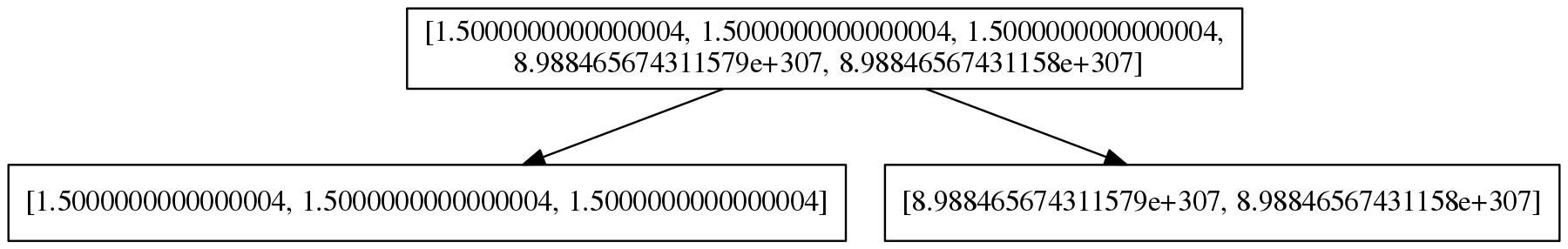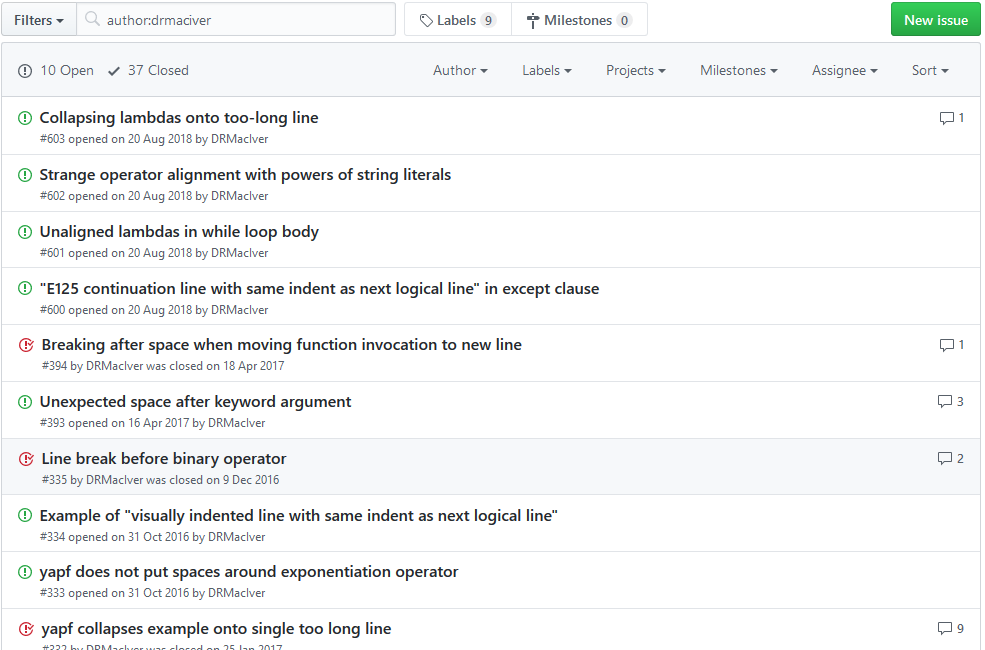# Can you make it smaller?

## what is test-case reduction?


$clang -O3 my_program.c -o my_program$ ./my_program
Segmentation Fault
$clang -O0 my_program.c -o my_program # time passes  it's never a compiler bug! ...except sometimes it is. (probably not with c though) it's a compiler bug! ...we should probably tell someone about it.  ... for (g_32 = (-26); (g_32 != 29); g_32++) { /* block id: 9 */ int8_t *l_68 = &g_69; uint8_t *l_75 = &g_76; uint32_t *l_81 = &g_82; const int32_t l_83 = 0xB46DFF61L; int32_t *l_84 = &g_85; int32_t l_87 = (-1L); int32_t *l_261 = (void *)0; uint16_t *l_277 = &g_180; int32_t *l_293 = {{&g_32, &g_32, &g_32, &g_32, &g_32, &g_32, &g_32}, {&l_87, &l_87, &l_87, &l_87, &l_87, &l_87, &l_87}, {&g_32, &g_32, &g_32, &g_32, &g_32, &g_32, &g_32}, {&g_32, &l_87, &g_32, &l_87, &g_32, &l_87, &g_32}}; int i, j; if (((*l_84) = (((*l_68) = p_63) && ((((safe_sub_func_uint32_t_u_u( ...   #include "stdint.h" int16_t a = 0; int32_t volatile b = 0; int32_t *c(uint8_t g, uint32_t h, uint16_t i) { j: goto j; } uint32_t d() { b; return 0; } int32_t e() { uint32_t f = {1}; c(a, d(), f); return 0; } int main() { e(); }  ## test-case reduction key idea: use small examples in bug reports. how do we get them? ## automated test-case reduction • an initial test case. • an interestingness test. • a test-case reducer. ## a test case • typically a file that triggers a bug • could be manual, often generated ## an interestingness test • typically "this test case triggers this bug" • can be quite tricky to write well! • more on this in a moment ## a test-case reducer • ☑ test case • ☑ test-case reducer • ☐ interestingness test  #!/usr/bin/env python import subprocess import sys COMPILER = "clang" RUNTIME = "/path/to/csmith-2.3.0/runtime/" if __name__ == '__main__': sourcefile = "my_program.c" target = "./my_program" try: compilation = subprocess.run( [COMPILER, sourcefile, "-O3", "-I", RUNTIME, "-o", target], timeout=5 ) if compilation.returncode != 0: sys.exit(1) result = subprocess.run(target, timeout=5) sys.exit(0 if result.returncode < 0 else 1) except subprocess.TimeoutExpired: sys.exit(1)  • ☑ test case • ☑ test-case reducer • ☑ interestingness test $ creduce ./is_interesting.py my_program.c


===< 62497 >===
running 4 interestingness tests in parallel
===< pass_unifdef :: 0 >===
(9.9 %, 91023 bytes)
===< pass_ifs :: 0 >===
===< pass_includes :: 0 >===
===< pass_line_markers :: 0 >===
===< pass_blank :: 0 >===
(10.0 %, 90954 bytes)
===< pass_clang_binsrch :: replace-function-def-with-decl >===
===< pass_clang_binsrch :: remove-unused-function >===
===< pass_lines :: 0 >===
(9.9 %, 91074 bytes)
(9.9 %, 91043 bytes)
...


#include "stdint.h"
a;
main() {
uint32_t b[] = {};
a = b;
}


$clang -I -O3 my_program.c -omy_program$ ./my_program
Illegal Instruction


## interestingness test problems

 slippage Getting a different bug than you wanted. validity Getting a test case that is allowed to be broken.

(secretly these are the same thing)

## brief digression: hypothesis


from hypothesis import given, strategies as st

def mean(ls):
return sum(ls) / len(ls)

@given(st.lists(st.floats(allow_nan=False, allow_infinity=False), min_size=1))
def test_mean(ls):
assert min(ls) <= mean(ls) <= max(ls)



Falsifying example: test_mean_is_within_bounds(
ls=[8.988465674311579e+307, 8.98846567431158e+307]
)

>   assert min(ls) <= mean(ls) <= max(ls)
E   assert inf <= 8.98846567431158e+307
E    +  where inf = mean([8.988465674311579e+307, 8.98846567431158e+307])
E    +  and   8.98846567431158e+307 = max([8.988465674311579e+307, 8.98846567431158e+307])


## interestingness test problems: validity


from hypothesis import given, strategies as st

def mean(ls):
return sum(ls) / len(ls)

@example([])
@given(st.lists(st.floats(allow_nan=False, allow_infinity=False), min_size=1))
def test_mean(ls):
assert min(ls) <= mean(ls) <= max(ls)


Falsifying example: test_mean_is_within_bounds(ls=[])

>       assert min(ls) <= mean(ls) <= max(ls)
E       ValueError: min() arg is an empty sequence



## interestingness test problems: slippage


from hypothesis import given, strategies as st

def mean(ls):
return sum(ls) / len(ls)

@given(st.lists(st.floats(allow_nan=False, allow_infinity=False), min_size=1))
def test_mean(ls):
assert min(ls) <= mean(ls) <= max(ls)



Falsifying example: test_mean_is_within_bounds(
ls=[1.5000000000000004, 1.5000000000000004, 1.5000000000000004]
)

>   assert min(ls) <= mean(ls) <= max(ls)
E   assert 1.5000000000000007 <= 1.5000000000000004
E    +  where 1.5000000000000007 = mean([1.5000000000000004, 1.5000000000000004, 1.5000000000000004])
E    +  and   1.5000000000000004 = max([1.5000000000000004, 1.5000000000000004, 1.5000000000000004])


## interestingness test problems: slippage## code compiles but executable crashes


#include "stdint.h"
a;
main() {
uint32_t b[] = {};
a = b;
}


$clang -I -O3 my_program.c -omy_program$ ./my_program
Illegal Instruction


## code compiles but executable segfaults


#include "csmith.h"
a;
b() {
c:
goto c;
}
d() {
int16_t *e = {};
for (; a; a = safe_add_func_int32_t_s_s);
}
main() {
d();
b();
}


## segfault despite undefined behaviour checking


#include "csmith.h"
int16_t a;
int32_t volatile b;
static int32_t *c(uint8_t, uint32_t, uint16_t);
static uint32_t d();
int32_t e() {
uint32_t f[] = {1};
c(a, d(), f);
return 0;
}
int32_t *c(uint8_t g, uint32_t h, uint16_t i) {
j:
goto j;
}
uint32_t d() {
b;
return 0;
}
int main() { e(); }


## manual tidying


#include "stdint.h"
int16_t a = 0;
int32_t volatile b = 0;
int32_t *c(uint8_t g, uint32_t h, uint16_t i) {
j:
goto j;
}
uint32_t d() {
b;
return 0;
}
int32_t e() {
uint32_t f = {1};
c(a, d(), f);
return 0;
}
int main() { e(); }


## shrink ray


#include "csmith.h"
volatile int g_792;
static int *func_2(uint8_t, uint32_t, uint16_t);
uint32_t func_12();
int func_1() {
int l = {};
func_2(
6, (((safe_div_func_uint64_t_u_u(
(safe_mod_func_uint32_t_u_u(
(func_12()), (safe_rshift_func_int16_t_s_u(
(((safe_rshift_func_int8_t_s_s) || 6)), l)))),
g_792)))),
l);
return (1);
}
int *func_2(uint8_t p3, uint32_t _, uint16_t p) {
lbl_1152: goto lbl_1152;
}
uint32_t func_12() { return 6; }
int main() { func_1(); }



## not just compilers!#!/usr/bin/env python

import subprocess, tempfile, sys, shutil
if __name__ == '__main__':
source = "source.py"
tmp = "tmp.py"
shutil.copyfile(source, tmp)
subprocess.check_call(["black", "-l79", tmp])
if subprocess.call(["pycodestyle", tmp]) != 0:
sys.exit(1)
subprocess.check_call(["yapf", "-i", "--style=pep8", tmp])
if subprocess.call(["pycodestyle", tmp]) == 0:
sys.exit(1)
sys.exit(0)


## not just compilers!

• language tools of all sorts
• data pipelines
• anything you can test!

## nb: imperative programming awaits


#!/usr/bin/env python

import os, sys, tempfile, subprocess

if __name__ == "__main__":
_, testfile, inputfile = sys.argv

with open(inputfile, "r") as i:

best = original

def test(s):
...


def test(s):
global best
with tempfile.TemporaryDirectory() as d:
with open(os.path.join(d, os.path.basename(inputfile)), "w") as o:
o.write(s)
try:
if subprocess.run(
os.path.abspath(testfile), cwd=d, timeout=5,
stdout=subprocess.DEVNULL, stderr=subprocess.DEVNULL,
).returncode == 0:
if len(s) < len(best):
print(f"Reduced best from {len(best)} to {len(s)} bytes")
best = s
with open(inputfile + ".reduced", "w") as o:
o.write(s)
return True
except subprocess.TimeoutExpired:
pass
return False
return result


def delete_lines():
print("Deleting lines")
lines = best.split("\n")

for i in range(len(lines) - 1, -1, -1):
attempt = lines[:i]+ lines[i + 1:]
if test('\n'.join(attempt)):
lines = attempt

prev = None
while prev != best:
prev = best

delete_lines()



$my_reducer ./is_interesting.py source.py   Deleting lines Deleting line 211 Reduced best from 8094 to 8093 bytes Deleting line 210 Deleting line 209 Reduced best from 8093 to 8041 bytes Deleting line 208 Deleting line 207 Reduced best from 8041 to 7977 bytes Deleting line 206 Reduced best from 7977 to 7901 bytes Deleting line 205 Reduced best from 7901 to 7858 bytes Deleting line 204 Reduced best from 7858 to 7803 bytes Deleting line 203 Reduced best from 7803 to 7754 bytes Deleting line 202 Deleting line 201 Deleting line 200 ...   WHEEL_NAME = re.compile( r"""^(?P<project_name>.+?)-(?P<version>\d.*?) )\.whl$""",
).match
NAMESPACE_PACKAGE_INIT = """\
"""

def unpack(src_dir, dst_dir):
for dirpath, dirnames, filenames in os.walk(src_dir):
for f in filenames:
os.renames(src, dst)
if match is None:
setattr(self, k, v)
return itertools.product()
for member in zf.namelist():
if dirname.endswith(".dist-info") and canonicalize_name(dirname).startswith(
canonicalize_name(self.project_name)
):
wheel_v1 = parse_version("1.0") <= wheel_version < parse_version("2.0dev0")
if not wheel_v1:
...


def indent_of(s):
length = len(s) - len(s.lstrip())
return s[:length]

def delete_indented_regions():
print("Deleting indented regions")
lines = best.splitlines()

i = 0
while i + 1 < len(lines):
indent = indent_of(lines[i])
j = i + 1
while j < len(lines) and (
not lines[j] or len(indent_of(lines[j])) > len(indent)
):
j += 1
if j > i + 1:
print(f"Deleting lines {i} to {j - 1}")
if j > i + 1 and test('\n'.join(lines[:i] + lines[j:])):
del lines[i:j]
else:
i += 1


prev = None
while prev != best:
prev = best

delete_indented_regions()

delete_lines()



\$ my_reducer ./is_interesting.py source.py


Deleting indented regions
Deleting lines 0 to 1
Reduced best from 8094 to 8070 bytes
Deleting lines 6 to 7
Reduced best from 8070 to 8054 bytes
...
Reduced best from 5033 to 4549 bytes
Deleting lines 17 to 73
Reduced best from 4549 to 2346 bytes
Deleting lines 18 to 44
...
Deleting lines 9 to 10
Reduced best from 452 to 313 bytes
Deleting lines
...
Deleting line 4
Deleting line 3
Reduced best from 313 to 254 bytes
Deleting line 2
...


NAMESPACE_PACKAGE_INIT = '''\
'''
class Wheel:
for subdir in filter(os.path.exists, (
os.path.join(dist_data, d)
for d in ('data', 'headers', 'purelib', 'platlib')
)):
unpack(subdir, destination_eggdir)